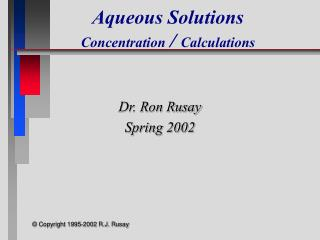# Aqueous Solutions Concentration / Calculations - PowerPoint PPT PresentationDownload PresentationAqueous Solutions Concentration / Calculations

Aqueous Solutions Concentration / Calculations
Download Presentation## Aqueous Solutions Concentration / Calculations

- - - - - - - - - - - - - - - - - - - - - - - - - - - E N D - - - - - - - - - - - - - - - - - - - - - - - - - - -
##### Presentation Transcript

1. Aqueous SolutionsConcentration / Calculations Dr. Ron Rusay Spring 2002 © Copyright 1995-2002 R.J. Rusay

2. Solutions • Homogeneous solutions are comprised of solute(s), the substance(s) dissolved, [The lesser amount of the component(s) in the mixture], and • solvent, the substance present in the largest amount. • Solutions with less solute dissolved than is physically possible are referred to as “unsaturated”. Those with a maximum amount of solute are “saturated”. • Occasionally there are extraordinary solutions that are “supersaturated” with more solute than normal.

3. Concentration and Temperature Relative Solution Concentrations: Saturated Unsaturated Supersaturated

4. DHMO, dihydromonoxide : “The Universal” Solvent http://www.dhmo.org

5. Water : “The Universal” Solvent The oil (nonpolar) and water (polar) mixture don’t mix and are immiscible. If liquids form a homogeneous mixture, they are miscible. Generally, likes dissolve likes, i.e. polar-polar and nonpolar-nonpolar. Polar and nonpolar do not mix , eg. oil and water

6. Aqueous Reactions & Solutions • Many reactions are done in a homogeneous liquid or gas phase which generally improves reaction rates. • The prime medium for many inorganic reactions is water which serves as a solvent (the substance present in the larger amount), but does not react itself. • The substance(s) dissolved in the solvent is (are) the solute(s). Together they comprise a solution. The reactants would be the solutes. • Reaction solutions typically have less solute dissolved than is possible and are “unsaturated”.

7. Salt dissolving in a glass of water

8. Solution Concentration • A solution’s concentration is the measure of the amount of solute dissolved. • Concentration is expressed in several ways. One way is mass percent. Mass % = Mass solute / [Mass solute + Mass solvent ] x100 • What is the mass % of 65.0 g of glucose dissolved in 135 g of water? Mass % = 65.0 g / [65.0 + 135]g x 100 = 32.5 %

9. Solution Concentration • Concentration is expressed more importantly as molarity (M). Molarity (M) = Moles solute / Liter Solution • An important relationship is M x Vsolution= mol • This relationship can be used directly in mass calculations of chemical reactions. • What is the molarity of a solution of 1.00 g KCl in 75.0 mL of solution? M KCl = [1.00g KCl / 75.0mL ][1molKCl / 74.55 g KCl ][ 1000mL / L] = 0.18 molKCl / L

10. Solution Concentration • The following formula can be used in dilution calculations: M1V1 = M2V2 • A concentrated stock solution is much easier to prepare and then dilute rather than preparing a dilute solution directly. Concentrated sulfuric acid is 18.0M. What volume would be needed to prepare 250.mL of a 1.50M solution? • V1 = M2V2 / M1 • V1 = 1.50 M x 250. mL / 18.0 M • V1 = 20.8 mL

11. Solution Applications • A solution of barium chloride was prepared by dissolving 26.0287 g in water to make 500.00 mL of solution. What is the concentration of the barium chloride solution? MBaCl2 = ? • MBaCl2= • =[26.0287g BaCl2 / 500.00mL ][1mol BaCl2 / 208.23g BaCl2 ] [1000mL / L] = 0.25000 mol / L

12. Solution Applications • 10.00 mL of this solution was diluted to make exactly 250.00 mL of solution which was then used to react with a solution of potassium sulfate. What is the concentration of the diluted solution. M2 = ? • MBaCl2= M1 • M2 = M1V1 / V2 • M2 = 0.25000 Mx 10.00 mL/ 250.00 mL • M2 = 0.010000 M

13. Solution Applications • 20.00 mL of the barium chloride solution required 15.50 mL of the potassium sulfate solution to react completely. MK2SO4 = ? • BaCl2(aq) + K2SO4(aq) ? + ? • BaCl2(aq) + K2SO4(aq) 2 KCl(aq) + BaSO4(s) • ?MK2SO4= [MBaCl2x VBaCl2 / VK2SO4 ] [? molK2SO4 / ? molBaCl2 ] • 0.010000 molBaCl2 x 0.02000 LBaCl2 x 1 molK2SO4 • LBaCl2x0.01550 LK2SO4x 1 molBaCl2 ?MK2SO4= ?MK2SO4= 0.01290 molK2SO4/ LK2SO4 = 0.01290 MK2SO4

14. ?gKCl = 0.010000 molBaCl2 / LBaCl2 x 0.02000 LBaCl2 X 2molKCl / 1molBaCl2 X 74.55 gKCl /molKCl Solution Applications How many grams of potassium chloride are produced? BaCl2(aq) + K2SO4(aq) ? + ? BaCl2(aq) + K2SO4(aq) 2 KCl(aq) + BaSO4(s) 1 = 0.02982gKCl

15. MolBaCl2 = MBaCl2x VBaCl2 =0.10molBaCl2 / LBaCl2x 0.02000LBaCl2 1 molBaCl2 = 2.0 x 10-3 MolK2SO4= MK2SO4x VK2SO4 =0.20molK2SO4 / LK2SO4x 0.01500LK2SO4 1 molK2SO4 = 3.0 x 10-3 Solution Applications If 20.00 mL of a 0.10 M solution of barium chloride was reacted with 15.00 mL of a 0.20 M solution of potassium sulfate, what would be the theoretical yield of barium sulfate? BaCl2(aq) + K2SO4(aq) 2 KCl(aq) + BaSO4(s) Which is the Limiting Reagent? 2.0 x 10-3< 3.0 x 10-3 2.0 x 10-3mol is limiting

16. Must use the limiting reagent: 0.10molBaCl2 x 0.02000LBaCl2 x1 molBaSO4 x 233.39 g BaSO4 LBaCl2 1 molBaCl2 molBaSO4 Solution Applications If 20.00 mL of a 0.10 M solution of barium chloride was reacted with 15.00 mL of a 0.20 M solution of potassium sulfate, what would be the theoretical yield of barium sulfate? BaCl2(aq) + K2SO4(aq) 2 KCl(aq) + BaSO4(s) = = 0.47 g